﻿ Space Equations: Time SeriesInternational Journal of Theoretical and Mathematical Physics

p-ISSN: 2167-6844    e-ISSN: 2167-6852

2019;  9(3): 63-66

doi:10.5923/j.ijtmp.20190903.01### Space Equations: Time Series

Prince Jessii

Trasker Institute of Scientific Research, 266 Main Rd, VV Mohalla, India

Correspondence to: Prince Jessii, Trasker Institute of Scientific Research, 266 Main Rd, VV Mohalla, India.
 Email: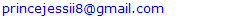Abstract

A progress from space equations which delivered the nature of space for planetary bodies in our solar system. This paper goes on to reveal the complete nature of space which is associated with time(space-time) and it presents a theory together with its equations to show how time moves according to the nature of space. The nature of space differs in each planetary body of mass and this results into different outcomes in each of the bodies in relation with time.

Keywords: Space, Time, Speed

Cite this paper: Prince Jessii, Space Equations: Time Series, International Journal of Theoretical and Mathematical Physics, Vol. 9 No. 3, 2019, pp. 63-66. doi: 10.5923/j.ijtmp.20190903.01.

### 1. Introduction

We can proceed by defining time as an indefinite continued progress of existence and events that occur in apparently irreversible succession from the past through the present to the future. Time can also be known as what a clock reads.
However, this means that time which is measured in seconds is continuous and it never stops. Lately, time was addressed with space to be part of a boundless four-dimensional continuum known as space-time, this means that space is associated with time, also that time can be defined from space.
We can know that an amount of time has passed through the help of the rotation and revolution of the earth which we used to mark time from a year, a day and down to seconds which a clock can measure.
Question: Lets say the earth was still, there were no external source of light (sun, moon and stars) that appears for a period of time, there are also no signs that reoccur to detect time on the atmosphere. How would we know that an amount of time has passed?
Answer: we can detect that an amount of time has passed when we suddenly see that our skin is fading and its not as fresh as it used to be.
Experimentally, if we have two newly born identical twins, we leave one on earth and we take the other to the moon. After several years, we’ll find out that one half of the twins which is on earth is aging faster than the other on the moon.
The reason is because of the difference in nature of space between the two planetary bodies.
However, time moves according to the nature of space, and the answer to the question which says: How is time related to space will be delivered at the end of this paper.

### 2. Discussion

In relating space with time, time can be in two forms which are: the normal time and the recreated time.
2.1. The Normal time: This is the time of the observer. It is the amount of time recorded by the observer’s clock concerning a particular event. Furthermore, the normal time is the time we all know.
2.2. The Recreated time: The recreated time exists through a time portal brought about by speed interacting with time. When a body is in motion with either a constant speed or a varying speed through space, a time portal opens, thereby recreating time. This time can only be dectected by a moving clock.
Futhermore, the recreated time is simply illustrated with this simple story.
Body of mass: Space, please I want to create my own time
Space: Oh really.
Space: Here’s the deal, I have a portal for you, the more speed you give me, the more time i’ll give you, and whenever you don’t use speed at all, I’ll close my portal so you can go back to your normal time.
Body of mass: Alright, Deal Accepted.
However, when a body in motion is observed, the total amount of time spent is given by:
Normal Time (tN) + Recreated Time (tR)

### 3. Methods & Results

Before the value of the magnitude of space in planets was found, objects with minimum & maximum speeds where moving through distances, but similarly we study their movement through space as related to distance. We know that moving through a distance is also moving through space, but in the past, we never thought moving through a distance with space would have effects probably because we don’t notice the effect due to a very free space here on earth.
We can address distance as speed x Time i.e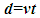(1)
If we replace distance, d with space, ﯼ in equation (1)
We have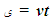(2)
This means that the associates of space are time and velocity and including gravity which we noticed in the previous paper (Reference ).
Note: The time involved in a motion through space is the recreated time, tR
Therefore, equation (2) changes to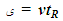(3)
Lets recall the table that shows the value of space for planetary bodies.
 Table 1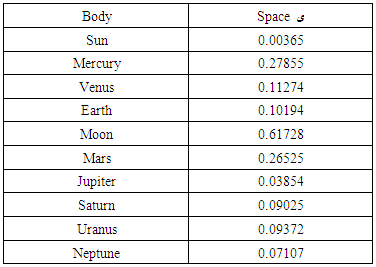Example 1: An observer on earth recorded an event which involves a moving body with an attached clock. The observer recorded 120sec for the moving body to reach its destination with a constant speed of 20m/s. What will be the total amount of time recorded by the moving clock?
Solution: we have v = 20m/s, tN=120sec, = 0.10194(the event was on earth).
Time portal created which leads to a recreated time tR = /v
(using equation ).
We have tR = 0.10194/20, tR = 0.0051sec.
Total time t recorded by the moving clock = tN + tR
We have 120sec + 0.0051sec. Total time t = 120.0051sec.
Lets look at the same situation on the moon.
Example 2: An observer on the moon recorded an event which involves a moving body with an attached clock. The observer recorded 120 sec for the moving body to reach its destination with a constant speed of 20m/s. What will be the total amount of time recorded by the moving clock?
Solution: we have v = 20m/s, tN=120sec, = 0.61728(the event was on the moon).
Time portal created which leads to a recreated time tR= /v
(using equation ).
We have tR= 0.61728/20, tR = 0.03sec.
Total time t recorded by the moving clock = tN + tR
We have 120sec + 0.03sec. Total time t = 120.03sec.
Comparing the two events recorded on different planetary bodies, with the total time, we observe that it took longer time for the body to reach its destination on the moon than on earth. This proves that space with a high magnitude slows down time, and that the higher the magnitude, the lesser time it takes. Moreover, we observe that, with the same speed on both planetary bodies, the moving body time travelled longer on the moon than on earth.
This results shows the outcome of time (recreated) in relation with speed interacting with space itself by inserting the different values of . Furthermore, we proceed to the outcome of time (normal) from any observer’s view with a stationary clock.
Physics equations in relation with space must involve at least one of the space associates.
All physics equations involving space changes outside the earth, equations like v= u +at, s =ut + 1/2at2, a=v/t etc.
To use such earth equation outside earth, we have to rewrite such equation according to the planet’s space.
Lets start with an equation of motion v=d/t, using an equation of motion outside the earth requires replacing one of the associates, lets use time as an example, the time it takes to reach a certain distance on earth is not the same in another planet.
Question: How can we calculate with the equations of motion on another planet?
Answer: PE = planet/ earth
For example, using PE = planet/earth with Mars, we have 0.26525/0.10194, therefore PE = 2.602021 for mars.
Table 2 shows PE calculated for planetary bodies in our solar system.
 Table 2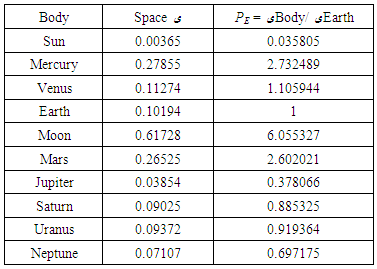To rewrite an equation of motion replacing time. we have to replace t with t/PE in equations of motion, table 3 shows how t will be replaced for planetary bodies.
Solving a problem which involves motion, for example
An object moves with a speed of 20m/s through a distance of 3000m. How long will it take the object to reach its destination, if the event was recorded for each of the following planets: earth,mars and venus.
Solution: we use equation v=d/t……(1)
in the problem above, d=3000m, v=20m/s.
for earth, we have t= d/v, 3000/20 =150sec
for mars, we have t/2.602021 =d/v, 3000/20= 150sec x 2.602021 = 390.30sec
for venus, we have t/1.105944=d/v, 3000/20 = 150sec
150sec x 1.105944=165sec.
Note: These values are neglecting air resistance.
 Table 3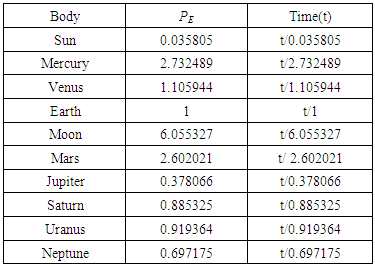With these, we can rewrite equation (1) as v= dPE/t…….(5).
Question: How can we use a physics equation for another planetary body?
Answer: we can use any physics equation for another planetary body by simply rewriting it according to space if it contains one of its associates.
Question: How can we rewrite a physics equation?.
Answer: The associates of space are three quantities which are time, velocity(speed) and gravity, but acceleration involves velocity and time only, this makes acceleration a space associate as part of velocity. So we have four associates i.e t,v,a and g.
However we can rewrite an equation according to space by simply replacing these quanties with its division with PE.
i.e a is replaced with a/PE , v is replaced with v/PE , t is replaced with t/PE and g is replaced with g/PE.
Note: if more than one of these three quantities are present in an equation, we have to pick just one of these three quantities and replace it according to space.
Table 4 shows some rewritten equations according to space which involves replacing only one of its associates in each equation. Furthermore, this proves that all fundamental and derived quantities changes in another different environment of space.
To summarize the results, to calculate g for another planet, we can simply take g for earth which is 9.8 and divide it by PE of the particular planet. E.g for venus we have 9.8/1.105944, we have 8.86m/s. This is how we truly know that gravity is related to space.
 Table 4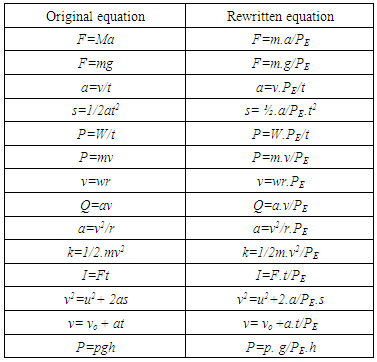Similarly for time, to calculate the exact time on another planet, we simply take time for earth, say 13:00am, we know that just one hour has passed from the start of the day.
Therefore one hour is equivalent to 3600sec.
To find the time in venus, we have t/PE which is 3600/1.105944, we have 3255sec. therefore, regardless of how we mark the rotation and revolution of a planet, we can say venus time is 345 secs slower or behind earth’s time at that moment.
Lastly, all theories in this paper are novel and originates from paper reference  and the following equations are proposed in this paper:
Total time= Normal Time (tN) + Recreated Time (tR)
PE = planet/ earth
= vtR
and how to rewrite a physics equation according to space using PE
These are all part of the space equations.

### 4. Conclusions

Space was the missing independent quantity in which all other quantities depends on it, which means all quantities must answer to space for their function.Time must always be defined with space (spacetime), therefore time is an indefinite continued progress of existence and events that occur through and in space in apparently irreversible succession from the past through the present to the future.
In conclusion, a verse from the holy book 2 Peter 3:8 says a day in heaven is like a thousand years on earth, who knows what the nature of space in heaven looks like?.

### ACKNOWLEDGEMENTS

I am grateful to GOD Almighty for revealing the idea used in creating the theory in this paper.

### References

  Prince Jessii, Space Equations, International Journal of Theoretical and Mathematical Physics, Vol. 9 No 2, 2019, pp. 41-44. doi: 10.5923/j.ijtmp.20190902.03.  Gamow, G., (2013) Gravity. Courier Corporation.  Time dilation, (1920) Albert Einstein.  Relativity [Uber die spezielle und die allgemeine Relativitatstheorie. Engl.], (1920) Albert Einstein.  Kaku, M., (2009) Physics of the Impossible.